## Principal Quintic Form

A general Quintic Equation(1)

can be reduced to one of the form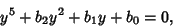(2)

called the principal quintic form.

Newton's Relations for the Rootsin terms of thes is a linear system in the, and solving for thes expresses them in terms of the Power sums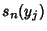. These Power sums can be expressed in terms of thes, so thes can be expressed in terms of thes. For a quintic to have no quartic or cubic term, the sums of the Roots and the sums of the Squares of the Roots vanish, so(3)(4)

Assume that the Rootsof the new quintic are related to the Rootsof the original quintic by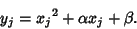(5)

Substituting this into (1) then yields two equations forand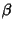which can be multiplied out, simplified by using Newton's Relations for the Power sums in the, and finally solved. Therefore,andcan be expressed using Radicals in terms of the Coefficients. Again by substitution into (4), we can calculate,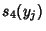andin terms ofandand the. By the previous solution forandand again by using Newton's Relations for the Power sums in the, we can ultimately express these Power sums in terms of the.